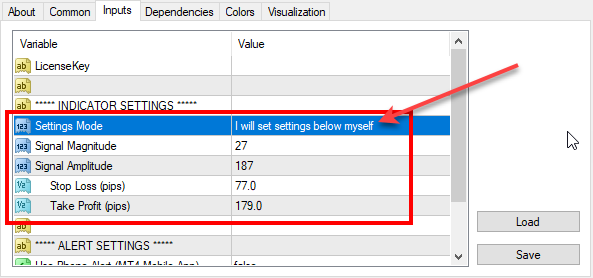# Re-optimized FxMagnetic indicator on GBPJPY (2019-10-07)

We’ve reoptimized FxMagnetic indicator settings on GBPJPY. Here are the latest parameters for all GBPJPY timeframes that you should use for best MT4 indicator performance.To set parameters manually you need to switch the Settings Mode to: I will set settings below myself

Don’t have FxMagnetic indicator yet? You can buy FxMagnetic here.

## 1-minute chart:

Signal Magnitude = 20

Signal Amplitude = 196

Stop Loss (pips) = 40

Take Profit (pips) = 125

## 5-minute chart:

Signal Magnitude = 60

Signal Amplitude = 217

Stop Loss (pips) = 39

Take Profit (pips) = 102

## 15-minute chart:

Signal Magnitude = 20

Signal Amplitude = 206

Stop Loss (pips) = 141

Take Profit (pips) = 243

## 30-minute chart:

Signal Magnitude = 98

Signal Amplitude = 222

Stop Loss (pips) = 94

Take Profit (pips) = 273

## 1-hour chart:

Signal Magnitude = 50

Signal Amplitude = 216

Stop Loss (pips) = 92

Take Profit (pips) = 237

## 4-hour chart:

Signal Magnitude = 30

Signal Amplitude = 164

Stop Loss (pips) = 237

Take Profit (pips) = 504

## Daily chart:

Signal Magnitude = 40

Signal Amplitude = 160

Stop Loss (pips) = 340

Take Profit (pips) = 550

## Weekly chart:

Signal Magnitude = 20

Ind0Param1 = 170

Stop Loss (pips) = 610

Take Profit (pips) = 720

## Monthly chart:

Signal Magnitude = 5

Signal Amplitude = 140

Stop Loss (pips) = 700

Take Profit (pips) = 2800

## FxMagnetic GBPJPY v1.2 stats on 7th October, 2019

Below are the screenshots of FxMagnetic GBPJPY stats from the previous 100 signals. This was taken on 2019-10-07 on the FxOpen Live ECN account. We re-optimized FxMagnetic GBPJPY v1.2 on this broker so you’ll see the best signals with them.

Remember, past performance does not guarantee future results.

We do not know the future and none of the world’s trading algorithms know the future. All the stats above and trading signals are based on pure mathematical formulas and probability theory.